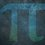# Transcendental-Natural Sequences

Definition: A sequence that has a transcendental number involved in the $n^{th}$ term and when $n$ is substituted and the result is rounded or left as it is, it produces all or most of the natural numbers.

Example:

$\frac{\pi}{4}$$+$ $\frac{x}{5}$$+$ $\frac{x^2}{69}$$+$ $\frac{x^3}{9168}$$+$ $\frac{x^4}{119999999}$

Result:

$1, 1, 1, 1, 2, 2, 3, 3, 4, 4, 5, 5, 6, 7, 7, 8, 9, 10, 10, 11, ...$

Challenge:

Prove that there is more sequences that meet the definition using algebraic proof or Python program.

Challenge $2$:

Prove that the sequence shows most of or all of the natural numbers using algebraic proof or Python program.Note by Yajat Shamji
10 months, 2 weeks ago

This discussion board is a place to discuss our Daily Challenges and the math and science related to those challenges. Explanations are more than just a solution — they should explain the steps and thinking strategies that you used to obtain the solution. Comments should further the discussion of math and science.

When posting on Brilliant:

• Use the emojis to react to an explanation, whether you're congratulating a job well done , or just really confused .
• Ask specific questions about the challenge or the steps in somebody's explanation. Well-posed questions can add a lot to the discussion, but posting "I don't understand!" doesn't help anyone.
• Try to contribute something new to the discussion, whether it is an extension, generalization or other idea related to the challenge.

MarkdownAppears as
*italics* or _italics_ italics
**bold** or __bold__ bold
- bulleted- list
• bulleted
• list
1. numbered2. list
1. numbered
2. list
Note: you must add a full line of space before and after lists for them to show up correctly
paragraph 1paragraph 2

paragraph 1

paragraph 2

[example link](https://brilliant.org)example link
> This is a quote
This is a quote
    # I indented these lines
# 4 spaces, and now they show
# up as a code block.

print "hello world"
# I indented these lines
# 4 spaces, and now they show
# up as a code block.

print "hello world"
MathAppears as
Remember to wrap math in $$ ... $$ or $ ... $ to ensure proper formatting.
2 \times 3 $2 \times 3$
2^{34} $2^{34}$
a_{i-1} $a_{i-1}$
\frac{2}{3} $\frac{2}{3}$
\sqrt{2} $\sqrt{2}$
\sum_{i=1}^3 $\sum_{i=1}^3$
\sin \theta $\sin \theta$
\boxed{123} $\boxed{123}$

Sort by:

@Yajat Shamji I wasn't able to see your comment in the report. What did you say?

- 10 months, 2 weeks ago

I deleted the report, that's why?

First comment was $11:30$am GMT?

Second comment was you really need proof (for the checkmate square), don't you?

- 10 months, 2 weeks ago

I have class exactly at that time. What about 2:30?

- 10 months, 2 weeks ago

GMT! Convert GMT to BST!

- 10 months, 2 weeks ago

3:30 pm

- 10 months, 2 weeks ago

$11:30$am GMT = $12:30$pm BST!

Seriously? You can't do that?

- 10 months, 2 weeks ago

Oh. I thought it was +1 in net. It is +13, then maybe we can't play at such time differences.

- 10 months, 2 weeks ago

In that case, I'll show you the checkmate square.

- 10 months, 2 weeks ago

- 10 months, 2 weeks ago

# Other sequences exist

A decreasing transcendental sequence can satisfy above equation as it is a decreasing function and difference will decrease between two consecutive numbers and will show all numbers eventually. @Yajat Shamji it's a challenge by me to find such function.

- 10 months, 2 weeks ago

All numbers don't exist in above sequence as

# Algebraic Proof

Above function is an increasing function, I.e. as we enter consecutive n's, the difference between consecutive values increase. At one point, the difference will go above 2 and one number won't exist.

# Code Proof

  1 2 3 4 5 6 7 8 9 10 11 12 13 14 15 16 17 18 19 20 21 22 23 a = [] n = 1000000 #enter number of natural numbers here for i in range(1, n + 1): a.append(0) for i in range(1, n + 1): b = int(3.14/4 + i/5 + (i * i)/69 + (i*i*i)/9168 + (i*i*i*i)/119999999) if b > n: break a[b - 1] = 1 flag = 1 for i in range(1, n + 1): if a[i] == 0: print("All numbers don't exist as %s doesn't exists" % i) flag = 0 break if flag == 1: print("All numbers exist") 

 1 2 3 Output: All numbers don't exist as 18 doesn't exists 

@Yajat Shamji

- 10 months, 2 weeks ago

For the second challenge, should I put doesn't? @Aryan Sanghi

And most in the definiton?

- 10 months, 2 weeks ago

Yes, you should put.

- 10 months, 2 weeks ago

Ok.

- 10 months, 2 weeks ago

Also, show the algebraic proof?

- 10 months, 2 weeks ago

I showed.

- 10 months, 2 weeks ago

Result?

- 10 months, 2 weeks ago

@Aryan Sanghi, I have made the note.

- 10 months, 2 weeks ago

Are you sure that whenever n is substituted, the number is transcendental in above equation?

- 10 months, 2 weeks ago

Yes. Should I add that? @Aryan Sanghi

- 10 months, 2 weeks ago

No, then it is ok.

- 10 months, 2 weeks ago

But what do you think, @Aryan Sanghi?

- 10 months, 2 weeks ago